MQL5 When Stop Loss is hit, wait for MACD to cross 0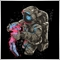95

Hi,

I have an EA working but I cannot seem to find a way to do the following:

1. Only have one trade at all times

2. When the stop loss is hit, wait for the MACD indicator to cross 0

Any advice/help would be amazing, I've been trying to find a way for weeks!

All the best,

Martin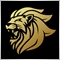Moderator
11487

`int ordersmax=1;`
```if(OrdersTotal()<ordersmax)
{
// Order...
}```

then combine.

```if(OrdersTotal()<ordersmax)
{
if(macd crossed 0)
{
// Order...
}
}```18873

Martin: Any advice/help would be amazing, I've been trying to find a way for weeks!
Help you with what? You haven't stated a problem, you stated a want. Show us your attempt (using CODE button) and state the nature of your problem.
No free help
urgent help.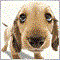156

Hi Martin,

the stop-loss is handled at the server side (the broker). You have no control over how it is handled, even not with an EA. When the stop loss is hit, the order will be closed, period. Although you may not realize it, or think this is not what you want, this is actually what stop loss is meant to do. It's a safeguard against al kinds of disasters that you, the EA or your internet connection might trigger.

What you probably want is a controlled exit out of a trade once a certain negative value is hit. Such a controlled exit should be handled on your trading platform (and not on the brokers server as a stop loss). Indeed, something you would use an EA for. If your EA doesn't support this then your options become limited (if it is an EA that you bought or downloaded).

If you are writing the EA yourself then you could do something like this (pseudo code):

1) Set a static variable:
bool low_limit_reached = false;
bool macd_cross_detected = false;

2) Keep track of the current profit (in pips) of your trade:
double profit = (CurrentPrice - OpenPrice)/Points (for SELL);

3) When profit falls below a certain treshhold, you set:
if (profit < -30.0) low_limit_reached = true;

4) Check if the MACD has crossed 0:
if (iMACD(,,,0) > 0 && iMACD(,,,1) < 0) macd_cross_detected = true; // once for SELL and once for BUY

5) Check if both conditions are true:
if (low_limit_detected && macd_cross_detected) OrderClose();

The code is very rough and just indicative of how you could solve it. But it should give you an idea on how to proceed.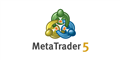is an instruction given to a broker to buy or sell a financial instrument. There are two main types of orders: Market and Pending. In addition, there are special Take Profit and Stop Loss levels. is the commercial exchange (buying or selling) of a financial security. Buying is executed at the demand price (Ask), and Sell is performed at the...95

Twan Jansbeken:

Hi Martin,

the stop-loss is handled at the server side (the broker). You have no control over how it is handled, even not with an EA. When the stop loss is hit, the order will be closed, period. Although you may not realize it, or think this is not what you want, this is actually what stop loss is meant to do. It's a safeguard against al kinds of disasters that you, the EA or your internet connection might trigger.

What you probably want is a controlled exit out of a trade once a certain negative value is hit. Such a controlled exit should be handled on your trading platform (and not on the brokers server as a stop loss). Indeed, something you would use an EA for. If your EA doesn't support this then your options become limited (if it is an EA that you bought or downloaded).

If you are writing the EA yourself then you could do something like this (pseudo code):

1) Set a static variable:
bool low_limit_reached = false;
bool macd_cross_detected = false;

2) Keep track of the current profit (in pips) of your trade:
double profit = (CurrentPrice - OpenPrice)/Points (for SELL);

3) When profit falls below a certain treshhold, you set:
if (profit < -30.0) low_limit_reached = true;

4) Check if the MACD has crossed 0:
if (iMACD(,,,0) > 0 && iMACD(,,,1) < 0) macd_cross_detected = true; // once for SELL and once for BUY

5) Check if both conditions are true:
if (low_limit_detected && macd_cross_detected) OrderClose();

The code is very rough and just indicative of how you could solve it. But it should give you an idea on how to proceed.

Thank you very much for your replies!

I have my stop losses already coded as well as the MACD cross (only for entries).

Here's my code, I added openlong and openshort booleans to detect whether a long/short has already been placed (in the "Try to open a deal" section. For example, if it enters a long, it will set openlong to true and will not take a long position until it opens a short. I want it to do this only when the stop loss is hit.

Any idea what I am missing?

All the best,

Martin

```//+------------------------------------------------------------------+
//|MartinStrategy.mq5 |

// M15 ONLY
// SL and TP 20-25 ONLY
// 50 EMA, 200 EMA, Stoch 49 3 3 work
// EMAs work
// Take profit works
// SL works

// ADD If SL is hit, wait until EMAs crosse again
// ADD "only one position at any time"

//+------------------------------------------------------------------+

input int  PositionSize           = 1;      // Position size (cost of one pip)
input int shortemaPeriods         = 50;
input int longemaPeriods          = 200;
input int stochastic_k_period     = 21;
input int stochastic_d_period     = 3;
input int stochastic_slowing_period =3;
input bool UseTakeProfit          = true;  // Use Take Profit
input int  Take_Profit            = 20;     // Take Profit
input int StopLossDistance        = 20;    // Stop loss distance in pips
input bool IsUseTrailingStop      = false;      //Use trailing stop
input int  TrailingStopDistance   = 40;    // Trailing stop distance in pips

bool openshort = false;
bool openlong  = false;

//---
double shortemaData[], longemaData[]; // You can declare multiple variables of the same data type in the same line.
int shortemaControlPanel, longemaControlPanel;
double shortema1, longema1;
int    stochastic_handle=0;
bool   ext_hedging=true;
CSymbolInfo symbol_info;
ulong arr_ticket_long_stochastic[];
ulong arr_ticket_long_rsi_stochastic[];
ulong arr_ticket_short_stochastic[];
ulong arr_ticket_short_rsi_stochastic[];
double trailing_stop_value;
double take_profit_value;
double stop_loss_value;
int tick_counter=0;
int time_in_open_function=-1;
int time_in_close_function=-1;

int Cnt;

#define MA_MAGIC 2719281928459450

//+------------------------------------------------------------------+
//| Expert initialization function                                   |
//+------------------------------------------------------------------+
int OnInit()
{

ArraySetAsSeries(shortemaData,true);   // Setting up table/array for time series data
ArraySetAsSeries(longemaData,true);    // Setting up table/array for time series data

shortemaControlPanel = iMA(_Symbol, PERIOD_M15, shortemaPeriods, 0, MODE_EMA, PRICE_CLOSE); // Getting the Control Panel/Handle for short SMA
longemaControlPanel = iMA(_Symbol, PERIOD_M15, longemaPeriods, 0, MODE_EMA, PRICE_CLOSE); // Getting the Control Panel/Handle for long SMA

//--- prepare trade class to control positions if hedging mode is active
ext_hedging=((ENUM_ACCOUNT_MARGIN_MODE)AccountInfoInteger(ACCOUNT_MARGIN_MODE)==ACCOUNT_MARGIN_MODE_RETAIL_HEDGING);
symbol_info.Name(_Symbol);

if(symbol_info.Digits()==3 || symbol_info.Digits()==5)

//initialize handle for stochastic
stochastic_handle=iStochastic(_Symbol,_Period,stochastic_k_period,stochastic_d_period,stochastic_slowing_period,MODE_SMA,STO_LOWHIGH);
if(stochastic_handle==INVALID_HANDLE)
{
printf("Error creating Stochastic oscillator");
return(INIT_FAILED);
}

ArrayResize(arr_ticket_long_stochastic,0,100000);
ArrayResize(arr_ticket_long_rsi_stochastic,0,100000);
ArrayResize(arr_ticket_short_stochastic,0,100000);
ArrayResize(arr_ticket_short_rsi_stochastic,0,100000);
//--- ok
return(INIT_SUCCEEDED);
}
//+------------------------------------------------------------------+
//| Expert deinitialization function                                 |
//+------------------------------------------------------------------+
void OnDeinit(const int reason)
{

IndicatorRelease(shortemaControlPanel);
IndicatorRelease(longemaControlPanel);

}
//+------------------------------------------------------------------+
//| Expert tick function                                             |
//+------------------------------------------------------------------+
void OnTick()
{
CheckForClose();
CheckForOpen();

numberOfShortemaData = CopyBuffer(shortemaControlPanel, 0, 0, 3, shortemaData); // Collect most current SMA(10) Data and store it in the datatable/array shortSmaData[]
numberOfLongemaData = CopyBuffer(longemaControlPanel, 0, 0, 3, longemaData); // Collect most current SMA(40) Data and store it in the datatable/array longSmaData[]

}

//+------------------------------------------------------------------+
//+------------------------------------------------------------------+
{
//---

int      Cnt;
ulong    Ticket;
datetime DT;

Cnt = 0;

if(CopyTime(_Symbol, PERIOD_M15, 0, 1, DT) <= 0)
{
Cnt = -1;
}
else
{
HistorySelect(DT, TimeCurrent());

for(int i = HistoryDealsTotal() - 1; i >= 0; i--)
{
Ticket = HistoryDealGetTicket(i);

if(HistoryDealGetString(Ticket, DEAL_SYMBOL) == _Symbol)
{
if(HistoryDealGetInteger(Ticket, DEAL_ENTRY) == DEAL_ENTRY_IN)
{
Cnt++;
}
}
}
}

//---
return(Cnt);
}

//+------------------------------------------------------------------+
//| Try to open the deal                                          |
//+------------------------------------------------------------------+
void CheckForOpen()
{
MqlRates rt;
if(CopyRates(_Symbol,_Period,0,2,rt)!=2)
{
Print("CopyRates of ",_Symbol," failed, no history");
return ;
}

datetime cur_time=rt.time;
MqlDateTime mdt;
TimeToStruct(cur_time,mdt);
int cur_calculated_time=mdt.hour*60+mdt.min;
if(cur_calculated_time==time_in_open_function)
return;
time_in_open_function=cur_calculated_time;
/*if(rt.tick_volume>1)
return ;*/

//remove unnecessary orders
RefreshOrders();
//move trailing stop orders if it is necessary
ModifyOrders();

//reserve array for Stochastic
double   stochastic_k_line;
double   stochastic_d_line;

//get Stochastic k-line values
if(CopyBuffer(stochastic_handle,MAIN_LINE,0,3,stochastic_k_line)!=3)
{
Print("CopyBuffer from iStochastic failed, no data");
return ;
}
//get Stochastic d-line values
if(CopyBuffer(stochastic_handle,SIGNAL_LINE,0,3,stochastic_d_line)!=3)
{
Print("CopyBuffer from iStochastic failed, no data");
return ;
}

string str_date_time= TimeToString(TimeCurrent())+" ";

//check condition "Stochastic indicator (%K line) enters the 0-20 zone"
//to open long position
if((shortema1 > longema1) && (openlong = false))
{
if(stochastic_k_line>20 && stochastic_k_line<=20)
{

openlong = true;
openshort = false;

Alert("Open long position, because k stochastic enters 0;20 zone");
Print("Try to open long for ",_Symbol);
return;
}
}

//check condition "Stochastic indicator (%K line) leaves the 80-100 zone"
//to open short position
if((shortema1 < longema1) && (openshort = false))
{
if(stochastic_k_line>80 && stochastic_k_line<=80)
{

openlong= false;
openshort = true;

Alert("Open short position, because k stochastic leaves 80;100 zone");
Print("Try to open short for ",_Symbol);
OpenPosition(arr_ticket_short_stochastic,ORDER_TYPE_SELL);
return;
}
}
}
//+------------------------------------------------------------------+
//| Open position                                                  |
//+------------------------------------------------------------------+
void OpenPosition(ulong& arr_ticket[],ENUM_ORDER_TYPE order_type)
{

{
string str_date_time= TimeToString(TimeCurrent())+" ";
double stop_loss=0;
double take_profit=0;
double local_stop_loss_value=IsUseTrailingStop ? trailing_stop_value:stop_loss_value;
double bid_price=SymbolInfoDouble(_Symbol,SYMBOL_BID);
//Calculate stop loss price
if(local_stop_loss_value!=0)
NormalizeDouble(bid_price-local_stop_loss_value,Digits());

take_profit=order_type==ORDER_TYPE_SELL ? NormalizeDouble(bid_price-take_profit_value,Digits()):

//Calculate lot size
double lot_size=GetLotSize();
string str_comment=_Symbol+_Period+TimeToString(TimeCurrent());
stop_loss,take_profit,str_comment);
if(is_open)
{
Print("Terminal opened the deal for ",_Symbol);
uint total=PositionsTotal();
for(uint i=0; i<total; i++)
{
string symbol=PositionGetSymbol(i);
string comment=PositionGetString(POSITION_COMMENT);
if(symbol==_Symbol && comment==str_comment)
{
ticket=PositionGetInteger(POSITION_TICKET);

}

}
int array_size=ArraySize(arr_ticket);
ArrayResize(arr_ticket,array_size+1);
arr_ticket[array_size]=ticket;

}
else
{
Print("Terminal could not open deal for ",_Symbol);
Print("Terminal error code= ",result_ret_code);
}
}

}
//+------------------------------------------------------------------+
//| Check for close position conditions                                                 |
//+------------------------------------------------------------------+
bool CheckForClose()
{
MqlRates rt;
if(CopyRates(_Symbol,_Period,0,2,rt)!=2)
{
Print("CopyRates of ",_Symbol," failed, no history");
return false;
}
datetime cur_time=rt.time;
MqlDateTime mdt;
TimeToStruct(cur_time,mdt);
int cur_calculated_time=mdt.hour*60+mdt.min;
if(cur_calculated_time==time_in_close_function)
return false;
time_in_close_function=cur_calculated_time;
/*if(rt.tick_volume>1)
return false;*/

//--- get current Stochastic
double   stochastic_k_line;
double   stochastic_d_line;
if(CopyBuffer(stochastic_handle,MAIN_LINE,0,3,stochastic_k_line)!=3)
{
Print("CopyBuffer from iStochastic failed, no data");
return false;
}
if(CopyBuffer(stochastic_handle,SIGNAL_LINE,0,3,stochastic_d_line)!=3)
{
Print("CopyBuffer from iStochastic failed, no data");
return false;
}

return false;
}

//+------------------------------------------------------------------+
//| Close position with the specified ticket                                                 |
//+------------------------------------------------------------------+
void ClosePosition(ulong& arr_tickets[])
{
string date_time_string=TimeToString(TimeCurrent())+" ";
for(int i=0;i<ArraySize(arr_tickets);i++)
{
ulong ticket=arr_tickets[i];

bool is_selected=PositionSelectByTicket(ticket);
if(is_selected)
{

{
if(!is_close)
{
Print("Terminal could not close the position for",_Symbol," the error Code=",result_ret_code);
}
}

}
else
{

}
ticket=0;

}
ArrayResize(arr_tickets,0,10000);
}
//+------------------------------------------------------------------+
//| Remove unnecessary orders from tickets array                                                 |
//+------------------------------------------------------------------+
void RefreshArray(ulong& arr_ticket[])
{
ulong buffer_array[];
ArrayResize(buffer_array,0,10000);
int count=0;
for(int i=0;i<ArraySize(arr_ticket);i++)
{
ulong ticket=arr_ticket[i];
bool is_selected=PositionSelectByTicket(ticket);
if(is_selected)
{
ArrayResize(buffer_array,count+1,10000);
buffer_array[count]=ticket;
count++;
}
}
if(ArraySize(buffer_array)!=ArraySize(arr_ticket))
{
ArrayResize(arr_ticket,ArraySize(buffer_array),10000);
ArrayCopy(arr_ticket,buffer_array,0,0,WHOLE_ARRAY);
}
}
//+------------------------------------------------------------------+
//| Remove unnecessary orders from all tickets array                                                 |
//+------------------------------------------------------------------+
void RefreshOrders()
{
RefreshArray(arr_ticket_long_stochastic);
RefreshArray(arr_ticket_long_rsi_stochastic);
RefreshArray(arr_ticket_short_stochastic);
RefreshArray(arr_ticket_short_rsi_stochastic);
}
//+------------------------------------------------------------------+
//| Get lot size for the deal                                                 |
//+------------------------------------------------------------------+
double GetLotSize()
{
double lot_step=SymbolInfoDouble(_Symbol,SYMBOL_VOLUME_STEP);
double min_lot=SymbolInfoDouble(_Symbol,SYMBOL_VOLUME_MIN);
double max_lot=SymbolInfoDouble(_Symbol,SYMBOL_VOLUME_MAX);
lot_size_for_deal=GetLotSize(lot_size_for_deal, lot_step,min_lot,max_lot);
return lot_size_for_deal;
}
//+------------------------------------------------------------------+
//| Get best fit lot size for the calculated one                                                 |
//+------------------------------------------------------------------+
double GetLotSize(double calculated_lot_size,double lot_size_step,double min_lot_size,double max_lot_size)
{
if(calculated_lot_size<min_lot_size)
return min_lot_size;
if(calculated_lot_size>max_lot_size)
return max_lot_size;
double cur_lot_size=lot_size_step;
double next_lot_size=cur_lot_size+lot_size_step;
do
{
if(calculated_lot_size>=cur_lot_size && calculated_lot_size<=next_lot_size)
{
if(calculated_lot_size==cur_lot_size)
return cur_lot_size;
if(calculated_lot_size==next_lot_size)
return next_lot_size;
if(calculated_lot_size-cur_lot_size<next_lot_size-calculated_lot_size)
return cur_lot_size;
else
return next_lot_size;
}
cur_lot_size=next_lot_size;
next_lot_size=cur_lot_size+lot_size_step;
}while(true);
}
//+------------------------------------------------------------------+
//| Move trailing stop if it is necessary for all orders                                                 |
//+------------------------------------------------------------------+
void ModifyOrders()
{
ModifyOrders(arr_ticket_long_stochastic);
ModifyOrders(arr_ticket_long_rsi_stochastic);
ModifyOrders(arr_ticket_short_stochastic);
ModifyOrders(arr_ticket_short_rsi_stochastic);
}
//+------------------------------------------------------------------+
//| Move trailing stop if it is necessary for all orders from array                                                |
//+------------------------------------------------------------------+
void ModifyOrders(ulong& arr_ticket[])
{

if(!IsUseTrailingStop || TrailingStopDistance==0)
return;
for(int i=0;i<ArraySize(arr_ticket);i++)
{
ulong ticket=arr_ticket[i];
bool is_selected=PositionSelectByTicket(ticket);
if(is_selected)
{
ENUM_POSITION_TYPE position_type=PositionGetInteger(POSITION_TYPE);
double open_price=PositionGetDouble(POSITION_PRICE_OPEN);
double current_stop_loss=PositionGetDouble(POSITION_SL);
double local_stop_loss;
double bid_price=SymbolInfoDouble(_Symbol,SYMBOL_BID);
{
if(bid_price-open_price>trailing_stop_value)
{
local_stop_loss=NormalizeDouble(bid_price-trailing_stop_value,Digits());
if(current_stop_loss<local_stop_loss)
{
}
}
}
else if(position_type==POSITION_TYPE_SELL)
{
{
if(current_stop_loss>local_stop_loss)
{
}

}
}

}
}

}

```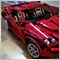547

Martin:

Thank you very much for your replies!

I have my stop losses already coded as well as the MACD cross (only for entries).

Here's my code, I added openlong and openshort booleans to detect whether a long/short has already been placed (in the "Try to open a deal" section. For example, if it enters a long, it will set openlong to true and will not take a long position until it opens a short. I want it to do this only when the stop loss is hit.

Any idea what I am missing?

All the best,

Martin

As @Twan Jansbeken mentioned, you'll have to keep track of your stop loss prices locally, without making calls to ext_trade.PositionModify in your ModifyOrders function.

Also, these lines contain bug and your openlong and openshort flags get set to false erroneously:

```   if((shortema1 > longema1) && (openlong = false))
:

if((shortema1 < longema1) && (openshort = false))
:```95

Seng Joo Thio:

As @Twan Jansbeken mentioned, you'll have to keep track of your stop loss prices locally, without making calls to ext_trade.PositionModify in your ModifyOrders function.

Also, these lines contain bug and your openlong and openshort flags get set to false erroneously:

Let me summarize what @Twan Jansbeken explained: it would close a position if open p&l is, say, -\$30 AND if the MACD crosses 0. Am I correct?

However, I want the EA to stop trading until the MACD crosses 0 again when the stop loss is hit. Wouldn't the openlong/openshort statement do the job?

I realized that my openlong and openshort are in the wrong place. They should be in the ModidyOrders function.

Also, I put the lines @Marco vd Heijden wrote around the following but it is not doing anything. I declared it as "int  ordermax  = 1" at the beginning of the code; Any ideas?

``` //to open long position
if(OrdersTotal()<ordermax)
{
if((shortema1 > longema1) && (openlong = false))
{
if(stochastic_k_line>20 && stochastic_k_line<=20)
{

openlong = true;
openshort = false;

Alert("Open long position, because k stochastic enters 0;20 zone");
Print("Try to open long for ",_Symbol);
return;
}
}

//check condition "Stochastic indicator (%K line) leaves the 80-100 zone"
//to open short position
if((shortema1 < longema1) && (openshort = false))
{
if(stochastic_k_line>80 && stochastic_k_line<=80)
{

openlong= false;
openshort = true;

Alert("Open short position, because k stochastic leaves 80;100 zone");
Print("Try to open short for ",_Symbol);
OpenPosition(arr_ticket_short_stochastic,ORDER_TYPE_SELL);
return;
}
}
}```547

Martin:

Let me summarize what @Twan Jansbeken explained: it would close a position if open p&l is, say, -\$30 AND if the MACD crosses 0. Am I correct?

However, I want the EA to stop trading until the MACD crosses 0 again when the stop loss is hit. Wouldn't the openlong/openshort statement do the job?

I realized that my openlong and openshort are in the wrong place. They should be in the ModidyOrders function.

Also, I put the lines @Marco vd Heijden wrote around the following but it is not doing anything. I declared it as "int  ordermax  = 1" at the beginning of the code; Any ideas?

Sorry, I misunderstood your requirements earlier. So ignore what I said about changing your ModifyOrders function with regard to stop loss settings.

Now, to do the stop trading until MACD crosses 0 part, I suggest instead of having openlong and openshort, just have one named MACDCut. Set it to true when (1) there is no trade, and (2) there's a zero crossing. Then open a new order only when MACDCut is true (plus your original stochastic conditions), but right after opening, set it to false.

And btw, double check this line - looks wrong:

`if(stochastic_k_line>80 && stochastic_k_line<=80)`95

Seng Joo Thio:

Sorry, I misunderstood your requirements earlier. So ignore what I said about changing your ModifyOrders function with regard to stop loss settings.

Now, to do the stop trading until MACD crosses 0 part, I suggest instead of having openlong and openshort, just have one named MACDCut. Set it to true when (1) there is no trade, and (2) there's a zero crossing. Then open a new order only when MACDCut is true (plus your original stochastic conditions), but right after opening, set it to false.

And btw, double check this line - looks wrong:

Thanks @Seng Joo Thio for pointing out the line, I corrected it.

The issue with this is that in a bullish MACD trend, for instance, the system will only take one position regardless of the number of long opportunities, until MACD crosses 0. I would like it to just stop trading after the stop loss was hit and then start again after a MACD zero cross.

Do I need to use HistoryDealSelect and then OnTradeTransaction?547

Martin:

Thanks @Seng Joo Thio for pointing out the line, I corrected it.

The issue with this is that in a bullish MACD trend, for instance, the system will only take one position regardless of the number of long opportunities, until MACD crosses 0. I would like it to just stop trading after the stop loss was hit and then start again after a MACD zero cross.

I'm thinking of have a line with if(OrderClosePrice() = OrderStopLoss()), then MACDcut = true. Is there a more efficient way?

This algorithm (same as what I described earlier) should suffice:

```        if (MACD Crosses zero && there is No On-going Trade)

MACDCut = true

if (MACDCut==true) {

MACDCut = false

}

if (Sell condition satisfied) {

MACDCut = false

}

}

// Do other stuff like trailing stops, etc.

```

Only slight tweaking, in future, if you decided to open more than one trade at one time...95

Seng Joo Thio:

This algorithm (same as what I described earlier) should suffice:

Only slight tweaking, in future, if you decided to open more than one trade at one time...

Yes but when the next stop loss is hit, it will continue opening trades until the MACD crosses 0 again.

That's what I am trying to do:

If Stop Loss is hit, then stop trading until MACD crosses 0 again.

Once the MACD crosses 0, MACDCut is reset.

Do you know how I can determine that the Stop Loss was hit?